Interactive online version:

# Bayesian Hierarchical Linear Regression¶

Author: Carlos Souza

Updated by: Chris Stoafer

Probabilistic Machine Learning models can not only make predictions about future data, but also model uncertainty. In areas such as personalized medicine, there might be a large amount of data, but there is still a relatively small amount of data for each patient. To customize predictions for each person it becomes necessary to build a model for each person — with its inherent uncertainties — and to couple these models together in a hierarchy so that information can be borrowed from other similar people .

The purpose of this tutorial is to demonstrate how to implement a Bayesian Hierarchical Linear Regression model using NumPyro. To motivate the tutorial, I will use OSIC Pulmonary Fibrosis Progression competition, hosted at Kaggle.

## 1. Understanding the task¶

Pulmonary fibrosis is a disorder with no known cause and no known cure, created by scarring of the lungs. In this competition, we were asked to predict a patient’s severity of decline in lung function. Lung function is assessed based on output from a spirometer, which measures the forced vital capacity (FVC), i.e. the volume of air exhaled.

In medical applications, it is useful to evaluate a model’s confidence in its decisions. Accordingly, the metric used to rank the teams was designed to reflect both the accuracy and certainty of each prediction. It’s a modified version of the Laplace Log Likelihood (more details on that later).

Let’s explore the data and see what’s that all about:

:

!pip install -q numpyro@git+https://github.com/pyro-ppl/numpyro arviz

:

import pandas as pd
import numpy as np
import seaborn as sns
import matplotlib.pyplot as plt

:

train = pd.read_csv(
"https://gist.githubusercontent.com/ucals/"
"2cf9d101992cb1b78c2cdd6e3bac6a4b/raw/"
"43034c39052dcf97d4b894d2ec1bc3f90f3623d9/"
"osic_pulmonary_fibrosis.csv"
)

:

Patient Weeks FVC Percent Age Sex SmokingStatus
0 ID00007637202177411956430 -4 2315 58.253649 79 Male Ex-smoker
1 ID00007637202177411956430 5 2214 55.712129 79 Male Ex-smoker
2 ID00007637202177411956430 7 2061 51.862104 79 Male Ex-smoker
3 ID00007637202177411956430 9 2144 53.950679 79 Male Ex-smoker
4 ID00007637202177411956430 11 2069 52.063412 79 Male Ex-smoker

In the dataset, we were provided with a baseline chest CT scan and associated clinical information for a set of patients. A patient has an image acquired at time Week = 0 and has numerous follow up visits over the course of approximately 1-2 years, at which time their FVC is measured. For this tutorial, I will use only the Patient ID, the weeks and the FVC measurements, discarding all the rest. Using only these columns enabled our team to achieve a competitive score, which shows the power of Bayesian hierarchical linear regression models especially when gauging uncertainty is an important part of the problem.

Since this is real medical data, the relative timing of FVC measurements varies widely, as shown in the 3 sample patients below:

:

def chart_patient(patient_id, ax):
data = train[train["Patient"] == patient_id]
x = data["Weeks"]
y = data["FVC"]
ax.set_title(patient_id)
sns.regplot(x=x, y=y, ax=ax, ci=None, line_kws={"color": "red"})

f, axes = plt.subplots(1, 3, figsize=(15, 5))
chart_patient("ID00007637202177411956430", axes)
chart_patient("ID00009637202177434476278", axes)
chart_patient("ID00010637202177584971671", axes)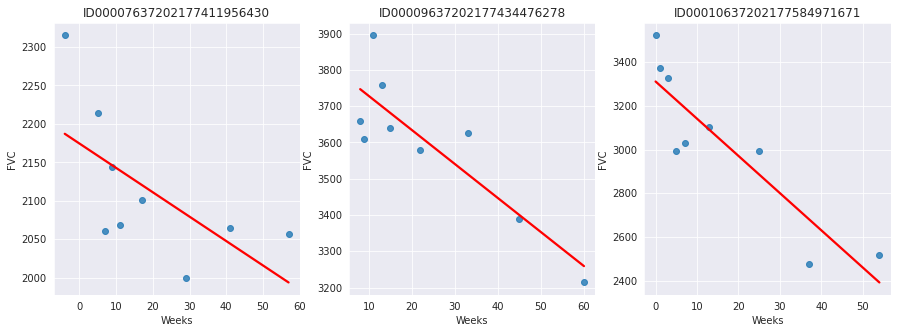On average, each of the 176 provided patients made 9 visits, when FVC was measured. The visits happened in specific weeks in the [-12, 133] interval. The decline in lung capacity is very clear. We see, though, they are very different from patient to patient.

We were are asked to predict every patient’s FVC measurement for every possible week in the [-12, 133] interval, and the confidence for each prediction. In other words: we were asked fill a matrix like the one below, and provide a confidence score for each prediction:The task was perfect to apply Bayesian inference. However, the vast majority of solutions shared by Kaggle community used discriminative machine learning models, disconsidering the fact that most discriminative methods are very poor at providing realistic uncertainty estimates. Because they are typically trained in a manner that optimizes the parameters to minimize some loss criterion (e.g. the predictive error), they do not, in general, encode any uncertainty in either their parameters or the subsequent predictions. Though many methods can produce uncertainty estimates either as a by-product or from a post-processing step, these are typically heuristic based, rather than stemming naturally from a statistically principled estimate of the target uncertainty distribution .

## 2. Modelling: Bayesian Hierarchical Linear Regression with Partial Pooling¶

The simplest possible linear regression, not hierarchical, would assume all FVC decline curves have the same $$\alpha$$ and $$\beta$$. That’s the pooled model. In the other extreme, we could assume a model where each patient has a personalized FVC decline curve, and these curves are completely unrelated. That’s the unpooled model, where each patient has completely separate regressions.

Here, I’ll use the middle ground: Partial pooling. Specifically, I’ll assume that while $$\alpha$$’s and $$\beta$$’s are different for each patient as in the unpooled case, the coefficients all share similarity. We can model this by assuming that each individual coefficient comes from a common group distribution. The image below represents this model graphically:Mathematically, the model is described by the following equations:

\begin{align} \mu_{\alpha} &\sim \mathcal{N}(0, 500) \\ \sigma_{\alpha} &\sim |\mathcal{N}(0, 100)| \\ \mu_{\beta} &\sim \mathcal{N}(0, 3) \\ \sigma_{\beta} &\sim |\mathcal{N}(0, 3)| \\ \alpha_i &\sim \mathcal{N}(\mu_{\alpha}, \sigma_{\alpha}) \\ \beta_i &\sim \mathcal{N}(\mu_{\beta}, \sigma_{\beta}) \\ \sigma &\sim \mathcal{N}(0, 100) \\ FVC_{ij} &\sim \mathcal{N}(\alpha_i + t \beta_i, \sigma) \end{align}

where t is the time in weeks. Those are very uninformative priors, but that’s ok: our model will converge!

Implementing this model in NumPyro is pretty straightforward:

:

import numpyro
from numpyro.infer import MCMC, NUTS, Predictive
import numpyro.distributions as dist
from jax import random

assert numpyro.__version__.startswith("0.10.0")

:

def model(patient_code, Weeks, FVC_obs=None):
μ_α = numpyro.sample("μ_α", dist.Normal(0.0, 500.0))
σ_α = numpyro.sample("σ_α", dist.HalfNormal(100.0))
μ_β = numpyro.sample("μ_β", dist.Normal(0.0, 3.0))
σ_β = numpyro.sample("σ_β", dist.HalfNormal(3.0))

n_patients = len(np.unique(patient_code))

with numpyro.plate("plate_i", n_patients):
α = numpyro.sample("α", dist.Normal(μ_α, σ_α))
β = numpyro.sample("β", dist.Normal(μ_β, σ_β))

σ = numpyro.sample("σ", dist.HalfNormal(100.0))
FVC_est = α[patient_code] + β[patient_code] * Weeks

with numpyro.plate("data", len(patient_code)):
numpyro.sample("obs", dist.Normal(FVC_est, σ), obs=FVC_obs)


That’s all for modelling!

## 3. Fitting the model¶

A great achievement of Probabilistic Programming Languages such as NumPyro is to decouple model specification and inference. After specifying my generative model, with priors, condition statements and data likelihood, I can leave the hard work to NumPyro’s inference engine.

Calling it requires just a few lines. Before we do it, let’s add a numerical Patient ID for each patient code. That can be easily done with scikit-learn’s LabelEncoder:

:

from sklearn.preprocessing import LabelEncoder

patient_encoder = LabelEncoder()
train["patient_code"] = patient_encoder.fit_transform(train["Patient"].values)

FVC_obs = train["FVC"].values
Weeks = train["Weeks"].values
patient_code = train["patient_code"].values


Now, calling NumPyro’s inference engine:

:

nuts_kernel = NUTS(model)

mcmc = MCMC(nuts_kernel, num_samples=2000, num_warmup=2000)
rng_key = random.PRNGKey(0)
mcmc.run(rng_key, patient_code, Weeks, FVC_obs=FVC_obs)

posterior_samples = mcmc.get_samples()

sample: 100%|██████████████████████████████████| 4000/4000 [00:51<00:00, 77.93it/s, 255 steps of size 1.48e-02. acc. prob=0.92]


## 4. Checking the model¶

### 4.1. Inspecting the learned parameters¶

First, let’s inspect the parameters learned. To do that, I will use ArviZ, which perfectly integrates with NumPyro:

:

import arviz as az

data = az.from_numpyro(mcmc)
az.plot_trace(data, compact=True, figsize=(15, 25));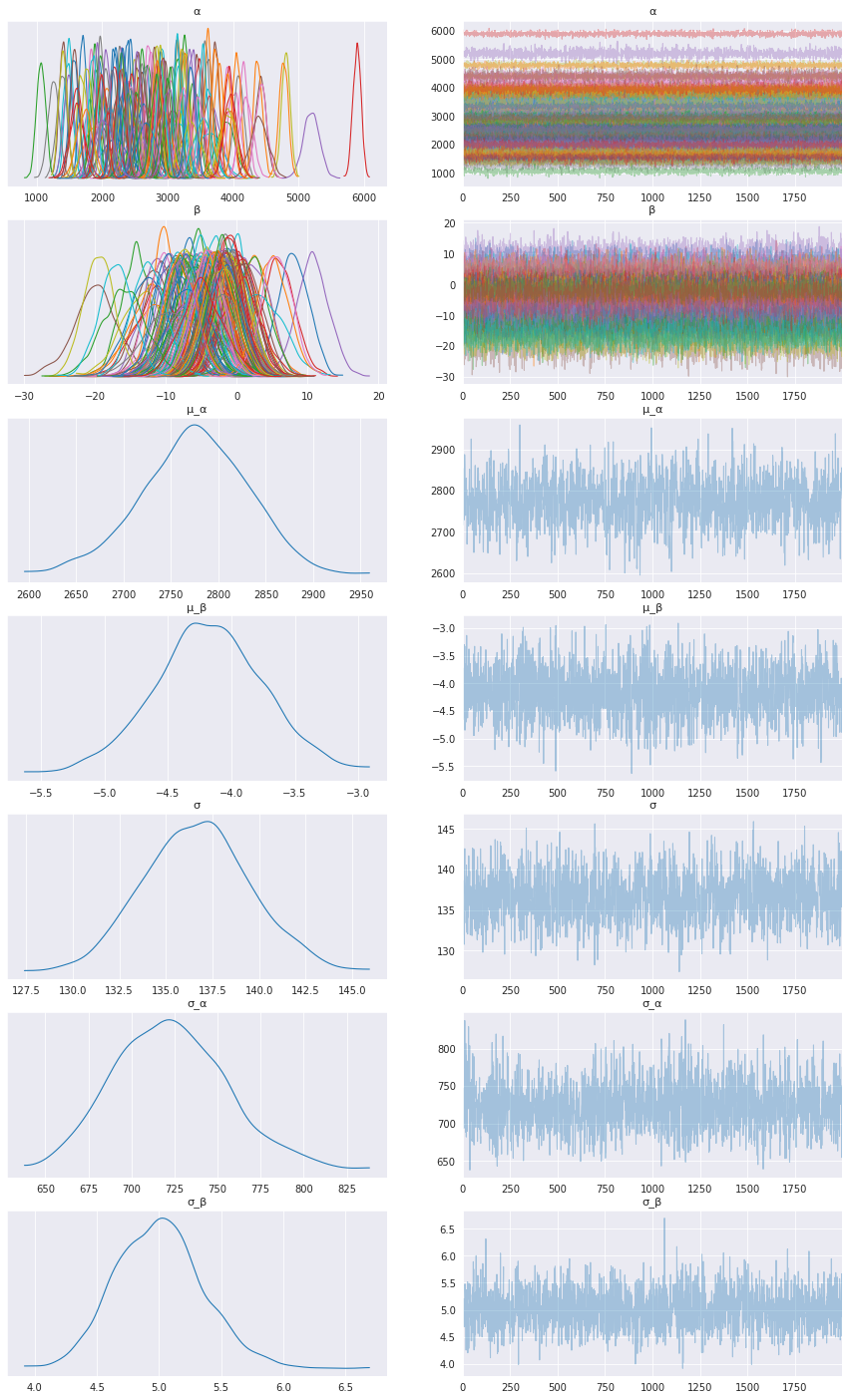Looks like our model learned personalized alphas and betas for each patient!

### 4.2. Visualizing FVC decline curves for some patients¶

Now, let’s visually inspect FVC decline curves predicted by our model. We will completely fill in the FVC table, predicting all missing values. The first step is to create a table to fill:

:

def create_prediction_template(unique_patient_df, weeks_series):
unique_patient_df["_temp"] = True
weeks = pd.DataFrame(weeks_series, columns=["Weeks"])
weeks["_temp"] = True
return unique_patient_df.merge(weeks, on="_temp").drop(["_temp"], axis=1)

:

patients = train[["Patient", "patient_code"]].drop_duplicates()
start_week_number = -12
end_week_number = 134
predict_weeks = pd.Series(np.arange(start_week_number, end_week_number))
pred_template = create_prediction_template(patients, predict_weeks)


Predicting the missing values in the FVC table and confidence (sigma) for each value becomes really easy:

:

patient_code = pred_template["patient_code"].values
Weeks = pred_template["Weeks"].values
predictive = Predictive(model, posterior_samples, return_sites=["σ", "obs"])
samples_predictive = predictive(random.PRNGKey(0), patient_code, Weeks, None)


Let’s now put the predictions together with the true values, to visualize them:

:

df = pred_template.copy()
df["FVC_pred"] = samples_predictive["obs"].T.mean(axis=1)
df["sigma"] = samples_predictive["obs"].T.std(axis=1)
df["FVC_inf"] = df["FVC_pred"] - df["sigma"]
df["FVC_sup"] = df["FVC_pred"] + df["sigma"]
df = pd.merge(
df, train[["Patient", "Weeks", "FVC"]], how="left", on=["Patient", "Weeks"]
)
df = df.rename(columns={"FVC": "FVC_true"})

:

Patient patient_code Weeks FVC_pred sigma FVC_inf FVC_sup FVC_true
0 ID00007637202177411956430 0 -12 2226.545166 160.158493 2066.386719 2386.703613 NaN
1 ID00007637202177411956430 0 -11 2216.172852 160.390778 2055.781982 2376.563721 NaN
2 ID00007637202177411956430 0 -10 2219.136963 155.339615 2063.797363 2374.476562 NaN
3 ID00007637202177411956430 0 -9 2214.727051 153.333313 2061.393799 2368.060303 NaN
4 ID00007637202177411956430 0 -8 2208.758545 157.368637 2051.389893 2366.127197 NaN

Finally, let’s see our predictions for 3 patients:

:

def chart_patient_with_predictions(patient_id, ax):
data = df[df["Patient"] == patient_id]
x = data["Weeks"]
ax.set_title(patient_id)
ax.plot(x, data["FVC_true"], "o")
ax.plot(x, data["FVC_pred"])
ax = sns.regplot(x=x, y=data["FVC_true"], ax=ax, ci=None, line_kws={"color": "red"})
ax.fill_between(x, data["FVC_inf"], data["FVC_sup"], alpha=0.5, color="#ffcd3c")
ax.set_ylabel("FVC")

f, axes = plt.subplots(1, 3, figsize=(15, 5))
chart_patient_with_predictions("ID00007637202177411956430", axes)
chart_patient_with_predictions("ID00009637202177434476278", axes)
chart_patient_with_predictions("ID00011637202177653955184", axes)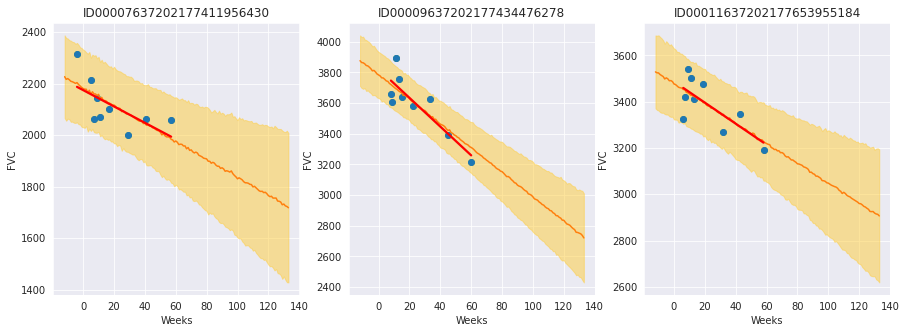The results are exactly what we expected to see! Highlight observations:

• The model adequately learned Bayesian Linear Regressions! The orange line (learned predicted FVC mean) is very inline with the red line (deterministic linear regression). But most important: it learned to predict uncertainty, showed in the light orange region (one sigma above and below the mean FVC line)

• The model predicts a higher uncertainty where the data points are more disperse (1st and 3rd patients). Conversely, where the points are closely grouped together (2nd patient), the model predicts a higher confidence (narrower light orange region)

• Finally, in all patients, we can see that the uncertainty grows as the look more into the future: the light orange region widens as the # of weeks grow!

### 4.3. Computing the modified Laplace Log Likelihood and RMSE¶

As mentioned earlier, the competition was evaluated on a modified version of the Laplace Log Likelihood. In medical applications, it is useful to evaluate a model’s confidence in its decisions. Accordingly, the metric is designed to reflect both the accuracy and certainty of each prediction.

For each true FVC measurement, we predicted both an FVC and a confidence measure (standard deviation $$\sigma$$). The metric was computed as:

\begin{align} \sigma_{clipped} &= max(\sigma, 70) \\ \delta &= min(|FVC_{true} - FVC_{pred}|, 1000) \\ metric &= -\dfrac{\sqrt{2}\delta}{\sigma_{clipped}} - \ln(\sqrt{2} \sigma_{clipped}) \end{align}

The error was thresholded at 1000 ml to avoid large errors adversely penalizing results, while the confidence values were clipped at 70 ml to reflect the approximate measurement uncertainty in FVC. The final score was calculated by averaging the metric across all (Patient, Week) pairs. Note that metric values will be negative and higher is better.

Next, we calculate the metric and RMSE:

:

y = df.dropna()
rmse = ((y["FVC_pred"] - y["FVC_true"]) ** 2).mean() ** (1 / 2)
print(f"RMSE: {rmse:.1f} ml")

sigma_c = y["sigma"].values
sigma_c[sigma_c < 70] = 70
delta = (y["FVC_pred"] - y["FVC_true"]).abs()
delta[delta > 1000] = 1000
lll = -np.sqrt(2) * delta / sigma_c - np.log(np.sqrt(2) * sigma_c)
print(f"Laplace Log Likelihood: {lll.mean():.4f}")

RMSE: 122.3 ml
Laplace Log Likelihood: -6.1406


What do these numbers mean? It means if you adopted this approach, you would outperform most of the public solutions in the competition. Curiously, the vast majority of public solutions adopt a standard deterministic Neural Network, modelling uncertainty through a quantile loss. Most of the people still adopt a frequentist approach.

Uncertainty for single predictions becomes more and more important in machine learning and is often a requirement. Especially when the consequences of a wrong prediction are high, we need to know what the probability distribution of an individual prediction is. For perspective, Kaggle just launched a new competition sponsored by Lyft, to build motion prediction models for self-driving vehicles. “We ask that you predict a few trajectories for every agent and provide a confidence score for each of them.”

## 5. Add layer to model hierarchy: Smoking Status¶

We can extend the model by including the column SmokingStatus as a pooling level, where model parameters will be partially pooled by the groups “Never smoked”, “Ex-smoker”, and “Currently smokes”. To do this, we need to:

1. Encode the SmokingStatus column

2. Map patient encoding to smoking status encodings

3. Refine and retrain the model with the additional hierarchy

:

train["SmokingStatus"].value_counts()

:

Ex-smoker           1038
Never smoked         429
Currently smokes      82
Name: SmokingStatus, dtype: int64

:

patient_code = train["patient_code"].values
Weeks = train["Weeks"].values

:

smoking_status_encoder = LabelEncoder()
train["smoking_status_code"] = smoking_status_encoder.fit_transform(
train["SmokingStatus"]
)

smoking_status_code = train["smoking_status_code"].values

:

map_patient_to_smoking_status = (
train[["patient_code", "smoking_status_code"]]
.drop_duplicates()
.set_index("patient_code", verify_integrity=True)
.sort_index()["smoking_status_code"]
.values
)

:

def model_smoking_hierarchy(
patient_code, Weeks, map_patient_to_smoking_status, FVC_obs=None
):
μ_α_global = numpyro.sample("μ_α_global", dist.Normal(0.0, 500.0))
σ_α_global = numpyro.sample("σ_α_global", dist.HalfNormal(100.0))
μ_β_global = numpyro.sample("μ_β_global", dist.Normal(0.0, 3.0))
σ_β_global = numpyro.sample("σ_β_global", dist.HalfNormal(3.0))

n_patients = len(np.unique(patient_code))
n_smoking_statuses = len(np.unique(map_patient_to_smoking_status))

with numpyro.plate("plate_smoking_status", n_smoking_statuses):
μ_α_smoking_status = numpyro.sample(
"μ_α_smoking_status", dist.Normal(μ_α_global, σ_α_global)
)
μ_β_smoking_status = numpyro.sample(
"μ_β_smoking_status", dist.Normal(μ_β_global, σ_β_global)
)

with numpyro.plate("plate_i", n_patients):
α = numpyro.sample(
"α",
dist.Normal(μ_α_smoking_status[map_patient_to_smoking_status], σ_α_global),
)
β = numpyro.sample(
"β",
dist.Normal(μ_β_smoking_status[map_patient_to_smoking_status], σ_β_global),
)

σ = numpyro.sample("σ", dist.HalfNormal(100.0))
FVC_est = α[patient_code] + β[patient_code] * Weeks

with numpyro.plate("data", len(patient_code)):
numpyro.sample("obs", dist.Normal(FVC_est, σ), obs=FVC_obs)


### Reparameterize the model¶

Hierarchical models often need to be reparameterized to enable MCMC to explore the full parameter space. NumPyro’s LocScaleReparam is used to do this below. For more details, see bad_posterior_geometry.ipynb and funnel.py. Thomas Wiecki also has a great post about developing non-centered models.

:

from numpyro.handlers import reparam
from numpyro.infer.reparam import LocScaleReparam

reparam_config = {
"μ_α_smoking_status": LocScaleReparam(0),
"μ_β_smoking_status": LocScaleReparam(0),
"α": LocScaleReparam(0),
"β": LocScaleReparam(0),
}
reparam_model_smoking_hierarchy = reparam(
model_smoking_hierarchy, config=reparam_config
)

:

nuts_kernel = NUTS(reparam_model_smoking_hierarchy, target_accept_prob=0.97)

mcmc = MCMC(nuts_kernel, num_samples=3000, num_warmup=5000)
rng_key = random.PRNGKey(0)
mcmc.run(rng_key, patient_code, Weeks, map_patient_to_smoking_status, FVC_obs=FVC_obs)

posterior_samples = mcmc.get_samples()

sample: 100%|█████████████████████████████████| 8000/8000 [03:55<00:00, 33.99it/s, 1023 steps of size 5.68e-03. acc. prob=0.97]


### 5.1. Inspect the learned parameters¶

:

data = az.from_numpyro(mcmc)
az.plot_trace(data, compact=True, figsize=(15, 45));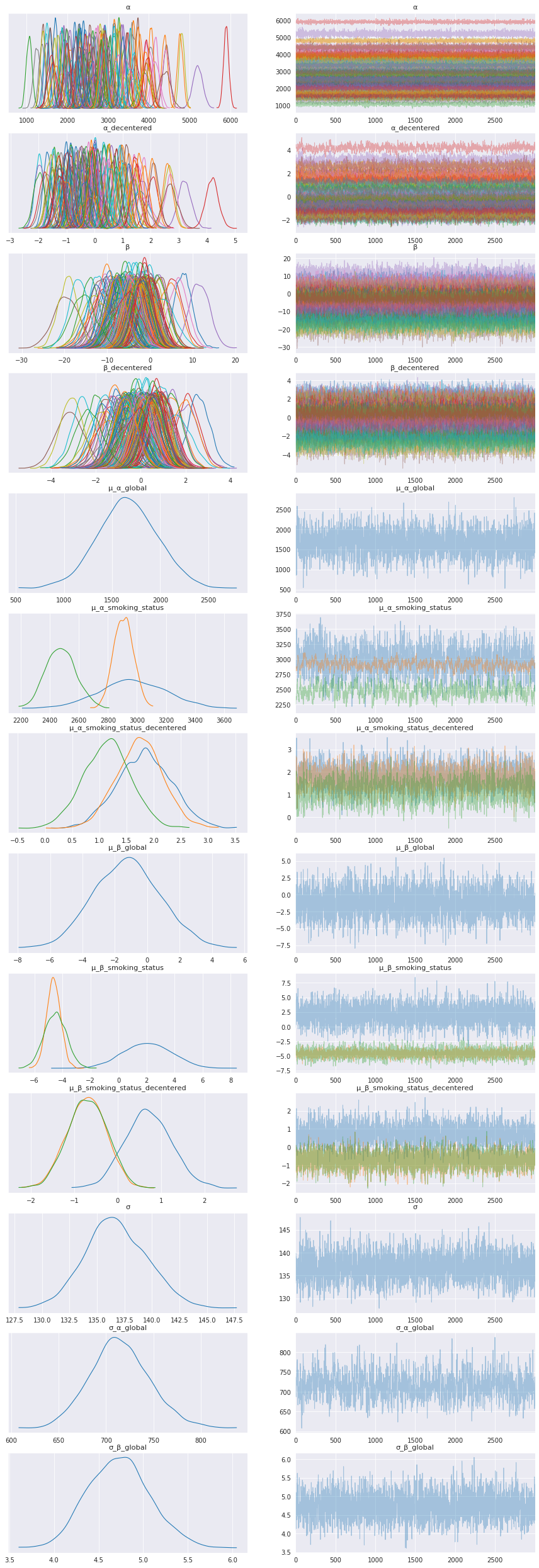#### Smoking Status distributions¶

Adding a legend for the smoking status distributions to help interpret the model results for that level.

Smoking Status

Code

Currently smokes

0

Ex-smoker

1

Never smoked

2

:

# Check the label code for each SmokingStatus
smoking_status_encoder.inverse_transform([0, 1, 2])

:

array(['Currently smokes', 'Ex-smoker', 'Never smoked'], dtype=object)

:

axes = az.plot_trace(
data,
var_names=["μ_α_smoking_status", "μ_β_smoking_status"],
legend=True,
compact=True,
figsize=(15, 15),
)
# The legend handles were not working for the first plot
axes[0, 0].legend();

WARNING:matplotlib.legend:No artists with labels found to put in legend.  Note that artists whose label start with an underscore are ignored when legend() is called with no argument.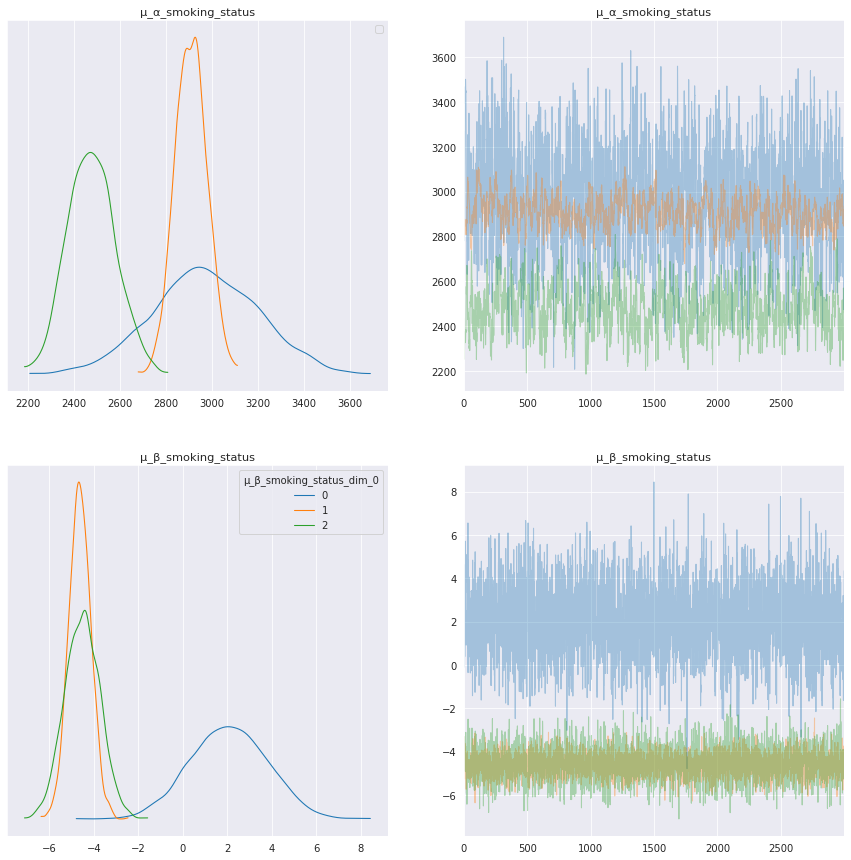### Interpret smoking status model parameters¶

The model parameters for each smoking status show interesting results, especially for trend, μ_β_smoking_status. In the trace plots above and summary table below the trend for current smokers, μ_β_smoking_status, has a positive mean, whereas the trend for patients that are ex-smokers and those that have never smoked are negative, μ_β_smoking_status and μ_β_smoking_status.

:

trace = az.from_numpyro(mcmc)
az.summary(
trace,
var_names=["μ_α_global", "μ_β_global", "μ_α_smoking_status", "μ_β_smoking_status"],
)

Shape validation failed: input_shape: (1, 3000), minimum_shape: (chains=2, draws=4)

:

mean sd hdi_3% hdi_97% mcse_mean mcse_sd ess_bulk ess_tail r_hat
μ_α_global 1660.172 309.657 1118.038 2274.933 6.589 4.660 2203.0 2086.0 NaN
μ_β_global -1.252 2.062 -5.014 2.678 0.037 0.035 3040.0 2041.0 NaN
μ_α_smoking_status 2970.486 227.761 2572.943 3429.343 7.674 5.452 878.0 1416.0 NaN
μ_α_smoking_status 2907.950 68.011 2782.993 3035.172 5.209 3.698 171.0 281.0 NaN
μ_α_smoking_status 2475.281 102.948 2286.072 2671.298 6.181 4.381 278.0 566.0 NaN
μ_β_smoking_status 2.061 1.713 -1.278 5.072 0.032 0.024 2797.0 2268.0 NaN
μ_β_smoking_status -4.625 0.498 -5.566 -3.721 0.010 0.007 2309.0 2346.0 NaN
μ_β_smoking_status -4.513 0.789 -6.011 -3.056 0.016 0.011 2466.0 2494.0 NaN

Let’s look at these curves for individual patients to help interpret these model results.

### 5.2. Visualizing FVC decline curves for some patients¶

:

patient_code = pred_template["patient_code"].values
Weeks = pred_template["Weeks"].values
predictive = Predictive(
reparam_model_smoking_hierarchy, posterior_samples, return_sites=["σ", "obs"]
)
samples_predictive = predictive(
random.PRNGKey(0), patient_code, Weeks, map_patient_to_smoking_status, None
)

:

df = pred_template.copy()
df["FVC_pred"] = samples_predictive["obs"].T.mean(axis=1)
df["sigma"] = samples_predictive["obs"].T.std(axis=1)
df["FVC_inf"] = df["FVC_pred"] - df["sigma"]
df["FVC_sup"] = df["FVC_pred"] + df["sigma"]
df = pd.merge(
df, train[["Patient", "Weeks", "FVC"]], how="left", on=["Patient", "Weeks"]
)
df = df.rename(columns={"FVC": "FVC_true"})

:

Patient patient_code Weeks FVC_pred sigma FVC_inf FVC_sup FVC_true
0 ID00007637202177411956430 0 -12 2229.098877 157.880753 2071.218018 2386.979736 NaN
1 ID00007637202177411956430 0 -11 2225.022461 157.358429 2067.664062 2382.380859 NaN
2 ID00007637202177411956430 0 -10 2224.487549 155.416016 2069.071533 2379.903564 NaN
3 ID00007637202177411956430 0 -9 2212.780518 154.162155 2058.618408 2366.942627 NaN
4 ID00007637202177411956430 0 -8 2219.202393 154.729507 2064.472900 2373.931885 NaN
:

f, axes = plt.subplots(1, 3, figsize=(15, 5))
chart_patient_with_predictions("ID00048637202185016727717", axes)  # Never smoked
chart_patient_with_predictions("ID00342637202287526592911", axes)  # Ex-smoker
chart_patient_with_predictions("ID00331637202286306023714", axes)  # Currently smokes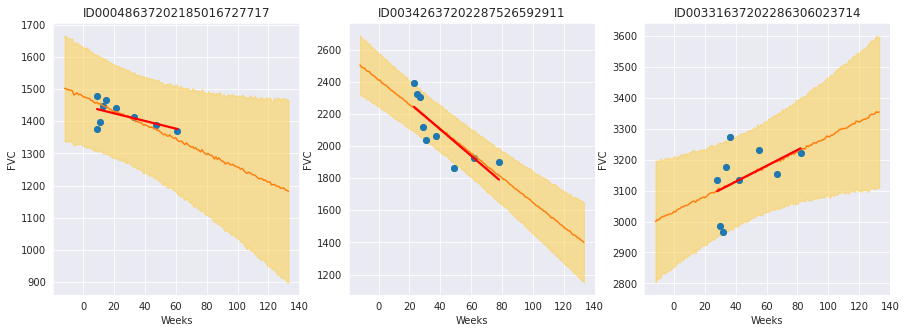### Review patients that currently smoke¶

By plotting each patient with the smoking status “Currently smokes”, we see some patients with a clear positive trend and others without a clear trend or negative trend. The trend lines are less overfit than the unpooled trend lines and show relatively large uncertainty in the slope and intercept. Depending on the model use case, we could proceed in different ways:

• If we just wanted to get an understanding of different attributes as they relate to patient’s FVC over time, we could stop here with an understanding that current smokers might have an increase in FVC over time when being monitored for Pulmonary Fibrosis. We might hypothesize causes for this observation to design a new experiment to test that hypothesis.

• If we wanted to develop a model that generates predictions used to treat patients, then we will want to make sure we are not overfitting so that we can trust the model with new patients. We might adjust model parameters to shrink the “Currently smokes” group model parameters to be closer to global parameters or even combine the group with “Ex-smokers”. We could look into collecting more data for current smokers to help ensure the model is not overfitting.

:

f, axes = plt.subplots(3, 3, figsize=(15, 15))
for i, patient in enumerate(
train[train["SmokingStatus"] == "Currently smokes"]["Patient"].unique()
):
chart_patient_with_predictions(patient, axes.flatten()[i])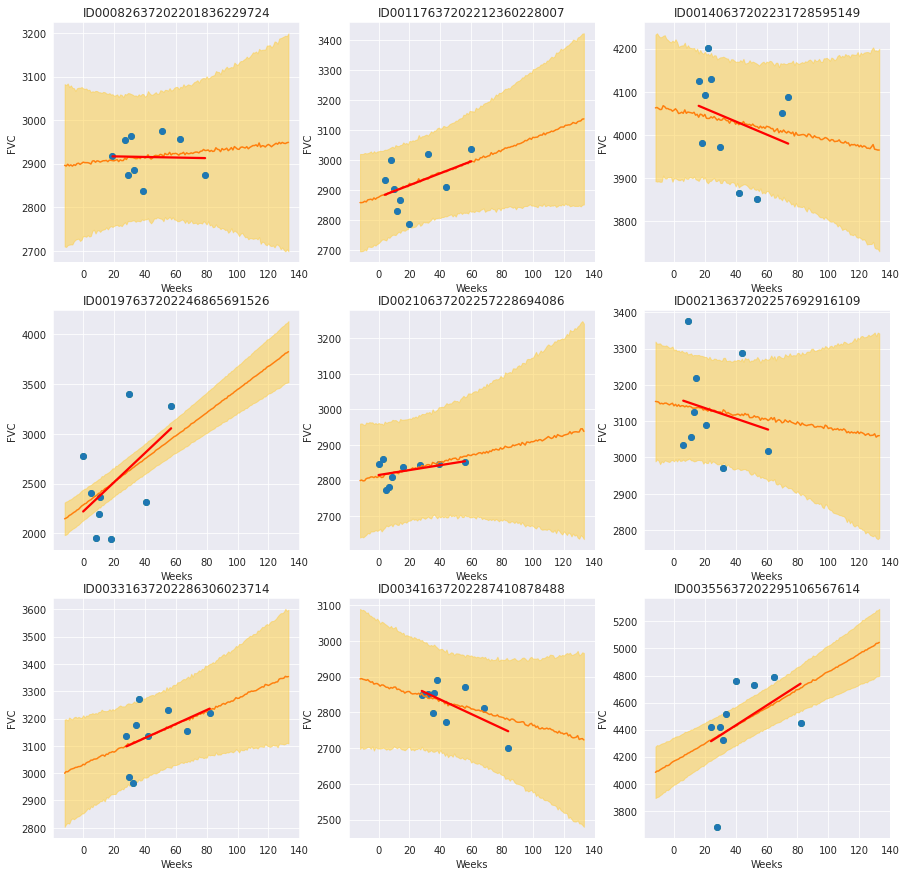### 5.3 Modified Laplace Log Likelihood and RMSE for model with Smoking Status Level¶

We calculate the metrics for the updated model and compare to the original model.

:

y = df.dropna()
rmse = ((y["FVC_pred"] - y["FVC_true"]) ** 2).mean() ** (1 / 2)
print(f"RMSE: {rmse:.1f} ml")

sigma_c = y["sigma"].values
sigma_c[sigma_c < 70] = 70
delta = (y["FVC_pred"] - y["FVC_true"]).abs()
delta[delta > 1000] = 1000
lll = -np.sqrt(2) * delta / sigma_c - np.log(np.sqrt(2) * sigma_c)
print(f"Laplace Log Likelihood: {lll.mean():.4f}")

RMSE: 122.6 ml
Laplace Log Likelihood: -6.1420


Both the Laplace Log Likelihood and RMSE show slightly worse performance for the smoking status model. We’ve learned that adding this hierarchy level as-is did not improve model performance, but we did find some interested results from the smoking status level that might be worth investigating. In addition, we might try to adjust priors or trying a different level (e.g. gender) to improve model performance.

## Wrap-up¶

Finally, I hope the great work done by Pyro/NumPyro developers help democratize Bayesian methods, empowering an ever growing community of researchers and practitioners to create models that can not only generate predictions, but also assess uncertainty in their predictions.